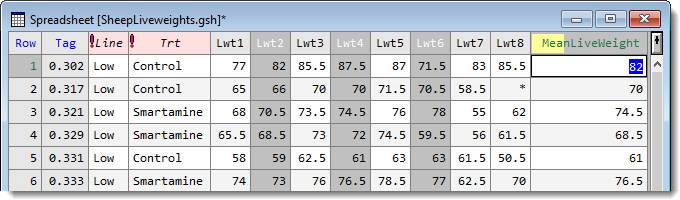1. Home
2. Summarizing Across Rows

# Summarizing Across Rows

You can calculate summaries across selected columns for each row in the spreadsheet. The summaries may be means, totals, minima, maxima, variances or standard deviations among others. If no columns are selected, you can summarize across all the columns or just columns of a certain type. The calculated results is inserted as a new column into your spreadsheet.

1. If you want to summarize a specific set of columns, pre-select these by holding down Ctrl while clicking the column headers.3. Select the rows to be used in forming summaries from the dropdown list.
4. Select the statistics to calculate.
5. Enter a name for the column containing the row summaries then click OK.

The resulting column is coloured half yellow to indicate that this is a calculated column.Columns to summarize

Lists the type of columns that can be used in forming the row summaries.

 All numeric columns All variate and factor columns within the spreadsheet. All variate columns All variate columns within the spreadsheet. All factor columns All factor columns within the spreadsheet. Selected columns The currently selected columns within the spreadsheet. This option is only available when a spreadsheet contains selected columns. Columns in pointer The columns belonging to a pointer defined within the spreadsheet. This option is only available when a spreadsheet contains pointers. Use the Spread | Sheet | Create pointers menu to define pointers in the spreadsheet.

Row summary to calculate

The following lists the statistics that can be calculated for each row along with the equivalent function that could be used to perform the same calculation using the CALCULATE directive in the command language.

 Statistic Description Genstat command Sum/Total The sum/total of the values in the row. VTOTALS/VSUMS Mean/Average The mean of the values in the row. VMEANS Standard deviation The standard deviation of the values in the row. VSD Variance The variance of the values in the row. VVARIANCE Standard error of means The standard error of the row mean. VSEMEANS Median The median value in the row. VMEDIANS Maxima The maximum value in the row. VMAXIMA Minima The minimum value in the row. VMINIMA Range The range of the values in the row. VRANGE No. of observations The number of non missing values. VNOBSERVATIONS Percentile The percentile of the values in the row (the percentage should be supplied in the space provided). VPERCENTILES Quantile The quantile of values in the row (the probability should be supplied in the space provided). VQUANTILES Position The position of a value in the row. The value to find in each row should be supplied in the space provided. This can be the name of another column in the spreadsheet or a number. VPOSITIONS No. of missing values The number of missing values (*). VNMV No. of values The number of columns. VNVALUES Skewness The skewness of the values. VSKEWNESS Kurtosis The kurtosis of the values. VKURTOSIS

Percentage (%) (for Summary type Percentile only)
A percentage between 0 and 100% for the calculation of the percentile. Percentages of 0, 25, 50 75, and 100 correspond to the minimum, lower quartile, median, upper quartile and maximum data value respectively.

Probability (for Summary type Quantile only)
A probability between 0 and 1 for the calculation of the quantile. Probabilities of 0, 0.25, 0.5, 0.75 and 1 correspond to the minimum, lower quartile, median, upper quartile and maximum data value respectively.

Find value (for Summary type Position only)
he value to find in each row. This can be the name of a variate or a number.

Save in
The column name to contain the row summaries.